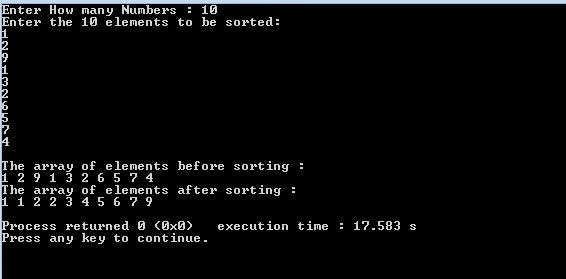# Bucket Sort algorithm implementation in C Programming

#### Bucket Sort:

Like Quicksort and insertion sort, there is another sort called Bucket sort. Bucket sort assumes that the input is drawn from a uniform distribution. Bucket sort runs in linear time on average, 0(n). It considers that the input is generated by a random process that distributes elements uniformly over the interval [0, 1].

#### Bucket sort works as follows:

•     Set up an array of initially empty buckets
•     Go over the original array, putting each object in its bucket.
•     Sort each non-empty bucket.
•     Visit the buckets in order and put all elements back into the original array.

Suppose we need to sort an array of positive integers.
A= {3,4,11,0,2,8,5,9,1,5,6,2,8,0,2,4,1,3,10,4,7,9}.

A bucket sort works as follows: create an array of size 11. Then, go through the input array and place integer 3 into a second array at index 3, integer 11 at index 11, and so on. We will end up with a sorted list in the second array.

#### Array A:

Moving through the array, we increment counters:

#### Array B:

Next, we simply read off the number of each occurrence:

#### Output:Output Screen

We can say Bucket sort is asymptotically fast (O(n) when the distribution is uniform). It is simple to code and suitable for a rough sort.